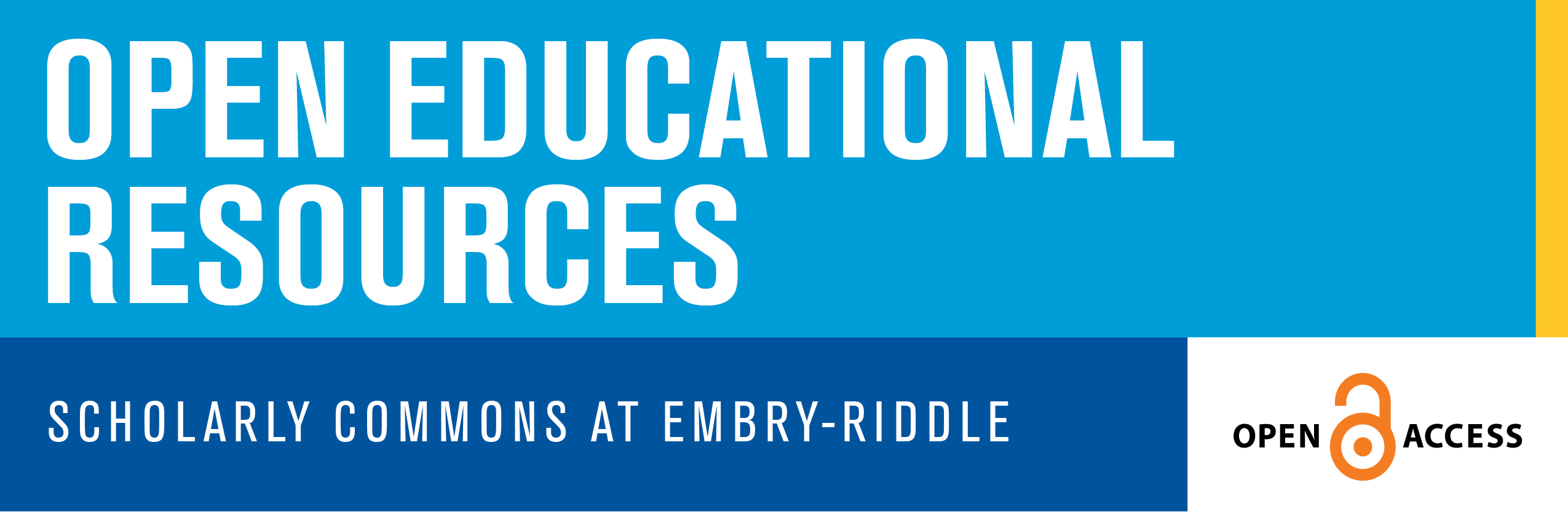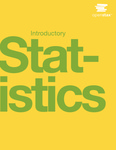## Open Access Textbooks

#### Title

Introductory Statistics

Book

#### Description

Introductory Statistics follows scope and sequence requirements of a one-semester introduction to statistics course and is geared toward students majoring in fields other than math or engineering. The text assumes some knowledge of intermediate algebra and focuses on statistics application over theory. Introductory Statistics includes innovative practical applications that make the text relevant and accessible, as well as collaborative exercises, technology integration problems, and statistics labs.

#### Contents

Preface

Chapter 1 Sampling and Data

Chapter 2 Descriptive Statistics

Chapter 3 Probability Topics

Chapter 4 Discrete Random Variables

Chapter 5 Continuous Random Variables

Chapter 6 The Normal Distribution

Chapter 7 The Central Limit Theorem

Chapter 8 Confidence Intervals

Chapter 9 Hypothesis Testing with One Sample

Chapter 10 Hypothesis Testing with Two Samples

Chapter 11 The Chi-Square Distribution

Chapter 12 Linear Regression and Correlation

Chapter 13 F Distribution and One-Way ANOVA

Appendix A: Review Exercises (Ch 3-13)

Appendix B: Practice Tests (1-4) and Final Exams

Appendix C: Data Sets

Appendix D: Group and Partner Projects

Appendix E: Solution Sheets

Appendix F: Mathematical Phrases, Symbols, and Formulas

Appendix G: Notes for the TI-83, 83+, 84, 84+ Calculators

Appendix H: Tables

Index

2018

#### Disciplines

Mathematics | Statistics and ProbabilityCOinS